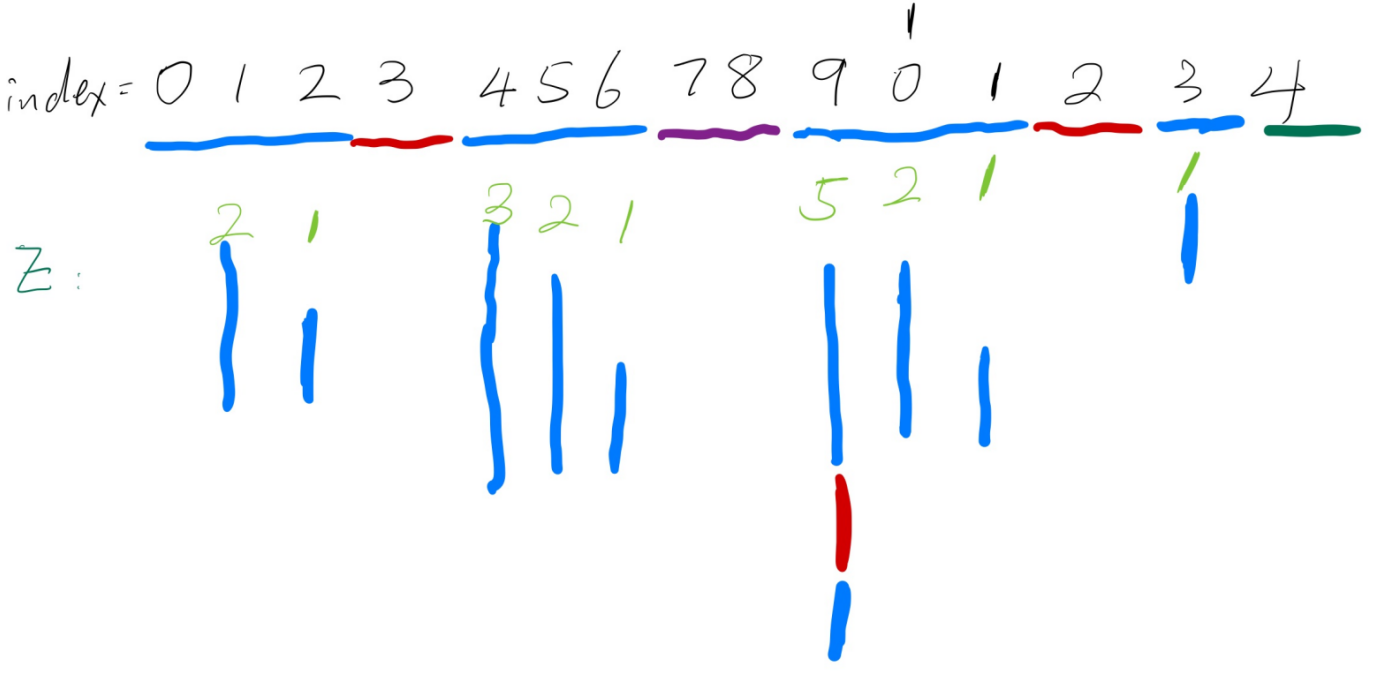#### 函数定义#### 线性时间求解

1. `L > 0`
2. `S[0, R - L] = S[L, R]`

L 与 R 均保持递增，易证算法的时间复杂度为 `O(n)`

`Z[i]` 时，

1. `i > R`，则这个 window 没有为我们提供已知的信息。令 `L = R = i`，再向右尽可能地延伸 R
2. `i <= R`，我们将 `S[L, R]` 拆分成 `S[L, i]``S[i, R]` (有意重叠`S[i]`)
• `k = i - L`. 因为 `S[0, R-L] = S[L, R]`， 我们可证 `S[0, k] = S[L, i]`
• 同理，`S[k, R - L] = S[i, R]`. 这样，我们就用上了 window 内的信息。
• 如果 `Z[k] < R - i + 1` ，就说明在子串 `S[k, R - L]` 中存在 `p >= Z[k]`, 使 `S[k+p] != S[0+p]`。 这也就是说， `S[i+p] != S[p]`。同样，对于任意 `q < Z[k]`，我们有 `S[i+q] = S[k+q] = S[q]`。因此，`Z[i] = Z[k]`
• 如果 `Z[k] >= R - i + 1`，我们在保持 L 不变的基础上，尽可能延伸 R

``````void extend_window(const char *str, int str_len, int left, int &right) {
// S[0:right-left] == S[left:right]   closed interval
// if S != S[right], resulting in  left+1==right, indicating an empty range
while (right < str_len && str[right - left] == str[right]) {
++right;
}
--right;
}
void z_function(const char *str, int str_len, int Z[]) {
Z = 0;
int left = -1;
int right = -1;
for (int i=1; i < str_len; ++i) {
if (i > right) {
left = right = i;
extend_window(str, str_len, left, right);
Z[i] = right - left + 1;
} else {
int k = i - left;
// We know S[0:right-left]  ==  S[left:right] =>
//      1. S[0:k]           ==  S[left:i]
//      2. S[k, right-left] ==  S[i:right]
if (Z[k] < right - i + 1) {
// exist p>=0, S[k+p] != S[p] => S[i+p] != S[p]
Z[i] = Z[k];
} else {
left = i;
extend_window(str, str_len, left, right);
Z[i] = right - left + 1;
}
}
}
}
``````

UT Dallas CS 6333 提供了一个直观的网页演示

#### 后记

Z Function 的英文定义可以参考 CMU 295 z-string-matching Page 7

e-maxx 提供了若干道例题，如 Codeforces - Password. Codechef 的教程也举出了两道例题。

resilar 提供了更为精简的实现

UT Dallas 的课程页面Matteo Dunnhofer 的文章 都提到了 Gusfield, Dan. Algorithms on Strings, Trees, and Sequences: Computer Science and Computational Biology. 但我手头并没有这本书，并没有验证 Gusfield 的书上是否提到了这一算法。